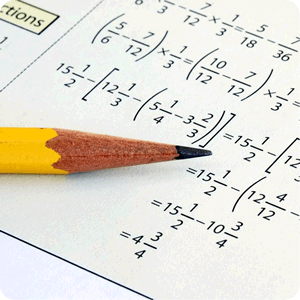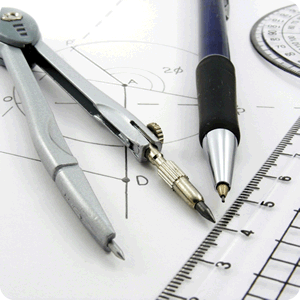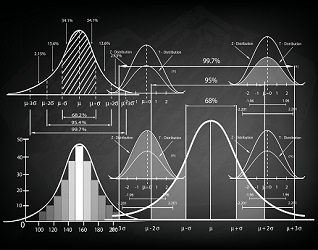Welcome to Free Math Help

What would you like to learn?Algebra

Covering pre-algebra through algebra 3 with a variety of introductory and advanced lessons.Geometry

Learn basic geometric shapes, properties, formulas, and problem solving techniques.Calculus

Look here for more advanced topics starting with pre-cal and extending through multivariable calculus.Statistics

Here's where we cover basics, like the mean & and median, along with links to more advanced statistics topics.Solvers

Solve your own math problems with our selection of free online calculator tools.